Published: 31 March 2018

# High performance estimations of natural frequency of basalt FRP laminated plates with intermediate elastic support using response surfaces method

Wael A. Altabey1
1International Institute for Urban Systems Engineering, Southeast University, Nanjing, 210096, China
1Nanjing Zhixing Information Technology Co., Ltd., Andemen Street, Nanjing, Jiangsu, China
1Department of Mechanical Engineering, Faculty of Engineering, Alexandria University, Alexandria, 21544, Egypt
Views 79

#### Abstract

Studying the changes of the natural frequencies due to intermediate elastic support of laminated composites is usually need a lot of computational processes or difficult to estimate. The present study employs a new high performance method for natural frequency estimating in basalt fiber reinforced polymer (FRP) laminated, variable thickness plates with intermediate elastic support based on the finite strip transition matrix (FSTM) with response surfaces (RS) method. Author has found that the FSTM method is very effective. However, a large error of estimation remains for estimation of natural frequency due to the large number of an iteration implemented in FSTM algorithm to estimate the natural frequency. In the present study, a new data processing procedure is proposed to improve performance of estimations of natural frequency. The estimation responses for four of classical boundary conditions at the plate ends with different elastic restraint coefficients (${K}_{T}$) are computed to obtain the first six frequency parameters ($\mathrm{\Omega }$). As a result, the method reveals excellent performance of estimations of natural frequencies.

## 1. Introduction

The classical and non-classical plates (i.e. Continuous and with intermediate stiffeners plates) are most common in a wide range of engineering fields such as aerospace, structures, vehicles and marine industries. In general, the approximate [1-10] or numerical [11-14] method must be used to compute the natural frequencies and find mode shapes for different boundary conditions for plate ends.

The author has investigated applicability of the FSTM method through a semi-analytical; natural frequency was estimated for basalt FRP laminated, variable thickness plates  and with intermediate elastic support. However, a large error of estimation remains due to a lot of computational processes must be performed for estimation of natural frequency. This is the main drawback of the FSTM method. Author also has already applied a predictive model for natural frequency in terms of fiber orientations is then developed using artificial neural networks (ANNs) to improve the estimations performance of FSTM by decreasing the effort to obtain frequency parameters in order to treatment the main drawback of the FSTM method . The developed model via ANNs is trained and tested with a number of the FSTM results then used this predictive model for predicting non- FSTM data not included in FSTM evaluation. An ANNs model is shown to be very effective for predicting the natural frequency of basalt FRP laminated, variable thickness plates with intermediate elastic support.

In light of those difficulties, the present study uses RS method of various elastic restraint coefficients (${K}_{T}$) of basalt FRP laminated, variable thickness plates with intermediate elastic support (Fig. 1) to investigate effect of intermediate elastic support on the natural frequencies; furthermore, an improvement to obtain higher estimation performance is proposed for the FSTM method before making response surfaces. This new method is applied to estimate natural frequencies of plate type under four different ends of the plate; performance of the new estimation method is evaluated analytically herein.

Fig. 1The geometrical model of Basalt FRP laminated variable thickness rectangular plate with intermediate elastic support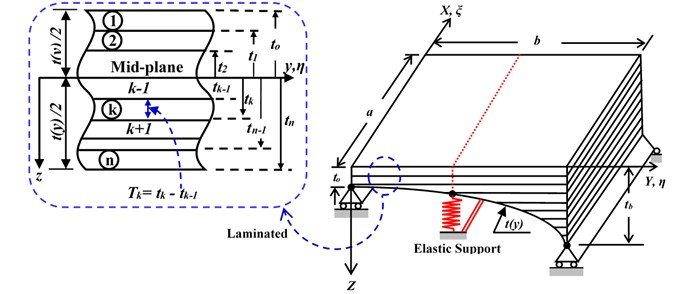## 2. Governing equations

The formula that governing the dynamics of presented plate model in this study using the classical deformation theory in terms of the plate deflection ${\delta }_{o}\left(x,y,\tau \right)$ after normalized to non-Dimensional variables $\xi$ and $\eta$ can be written as: [15, 17]:

1
${\varphi }_{1}\frac{1}{{a}^{4}}{\delta }_{\xi \xi \xi \xi }+\frac{2{\varphi }_{2}}{{t}^{3}\left(\eta \right)}\frac{1}{{a}^{2}b}\frac{\partial {t}^{3}\left(\eta \right)}{\partial \eta }{\delta }_{\xi \xi \eta }+2{\varphi }_{2}\frac{1}{{a}^{2}{b}^{2}}{\delta }_{\xi \xi \eta \eta }+{\varphi }_{3}\frac{1}{{a}^{3}b}{\delta }_{\xi \xi \xi \eta }+4{\varphi }_{4}\frac{1}{a{b}^{3}}{\delta }_{\xi \eta \eta \eta }$
$+\frac{1}{ab}\frac{4{\varphi }_{4}}{{t}^{3}\left(\eta \right)}\frac{{\partial }^{2}{t}^{3}\left(\eta \right)}{\partial {\eta }^{2}}{\delta }_{\xi \eta }+\frac{8{\varphi }_{4}}{{t}^{3}\left(\eta \right)}\frac{1}{a{b}^{2}}\frac{\partial {t}^{3}\left(\eta \right)}{\partial \eta }{\delta }_{\xi \eta \eta }+\frac{1}{{b}^{2}}\frac{1}{{t}^{3}\left(\eta \right)}\frac{{\partial }^{2}{t}^{3}\left(\eta \right)}{\partial {\eta }^{2}}{\delta }_{\eta \eta }+\frac{1}{{b}^{4}}{\delta }_{\eta \eta \eta \eta }$
$+\frac{2}{{t}^{3}\left(\eta \right)}\frac{1}{{b}^{3}}\frac{\partial {t}^{3}\left(\eta \right)}{\partial \eta }{\delta }_{\eta \eta \eta }=-\frac{{m}_{o}}{{D}_{22}}\frac{{t}_{o}^{2}}{{t}^{2}\left(\eta \right)}{\delta }_{\tau \tau },$

where: $\beta =a/b$ is the aspect ratio, and:

$\xi =\frac{x}{a},\eta =\frac{y}{b},{\varphi }_{1}=\frac{{D}_{11}}{{D}_{22}},{\varphi }_{2}=\frac{\left({D}_{12}+2{D}_{66}\right)}{{D}_{22}},{\varphi }_{3}=\frac{{D}_{16}}{{D}_{22}},{\varphi }_{4}=\frac{{D}_{26}}{{D}_{22}},$
${\delta }_{\xi \xi \xi \xi }=\frac{{\partial }^{4}{\delta }_{o}}{\partial {\xi }^{4}},{\delta }_{\eta \eta \eta \eta }=\frac{{\partial }^{4}{\delta }_{o}}{\partial {\eta }^{4}},{\delta }_{\xi \xi \eta \eta }=\frac{{\partial }^{4}{\delta }_{o}}{\partial {\xi }^{2}\partial {\eta }^{2}},{\delta }_{\xi \xi \xi \eta }=\frac{{\partial }^{4}{\delta }_{o}}{\partial {\xi }^{3}\partial \eta },$
${\delta }_{\eta \eta \eta \xi }=\frac{{\partial }^{4}{\delta }_{o}}{\partial {\eta }^{3}\partial \xi },{\delta }_{\tau \tau }=\frac{{\partial }^{2}{\delta }_{o}}{\partial {\tau }^{2}},{m}_{o}=\rho {t}_{o},$

$\rho$ is the material density, ${D}_{ij}$ is the flexural rigidities matrix are present by:

2
${D}_{ij}=\frac{1}{3}\frac{{t}^{3}\left(y\right)}{{t}_{o}^{3}}{\sum }_{k=1}^{n}{\left[\left({\overline{q}}_{ij}\right)\right]}_{k}\left({t}_{ok}^{3}-{t}_{ok-1}^{3}\right),i,j=1,2,3,\dots ,$

where ${t}_{ok}$ as shown in Fig. 1 is measuring between the middle-plane approbating to ${t}_{o}$ to the layer bottom ${t}_{oth}$. And $\overline{{q}_{ij}^{k}}$ are the plane stress transformed reduced stiffness coefficients, they can be computed in terms of the longitudinal and transverse Young’s moduli ${E}_{11}$, ${E}_{22}$, plane shear modulus of elasticity ${G}_{12}$ and Poisson coefficients ${\upsilon }_{12}$ and ${\upsilon }_{21}$ as:

3
${q}_{ij}=\left[\begin{array}{lll}{q}_{11}& {q}_{12}& {q}_{13}\\ {q}_{12}& {q}_{22}& {q}_{23}\\ {q}_{13}& {q}_{23}& {q}_{66}\end{array}\right]=\left[\begin{array}{ccc}\frac{{E}_{11}}{\left(1-{\upsilon }_{12}{\upsilon }_{21}\right)}& \frac{{\upsilon }_{21}{E}_{11}}{\left(1-{\upsilon }_{12}{\upsilon }_{21}\right)}& 0\\ \frac{{\upsilon }_{21}{E}_{11}}{\left(1-{\upsilon }_{12}{\upsilon }_{21}\right)}& \frac{{E}_{22}}{\left(1-{\upsilon }_{21}{\upsilon }_{12}\right)}& 0\\ 0& 0& {G}_{12}\end{array}\right].$

### 2.1. Boundary conditions

In this study, author applied new method to estimate natural frequencies of plate type under four different classical boundary conditions at the ends of the plate are SSSS, CCCC, SSFF and CCFF (see Fig. 2).

Since the treatment of the intermediate elastic line support conditions are the goal of the present study we can see in Fig. 1, the intermediate elastic line support, $y=b/2$ , the solution of the governing must be continuous at this line, i.e. :

4
$\frac{1}{b}{\frac{\partial {\delta }_{o}}{\partial \eta }|}_{\eta ={1}^{-}/2}=\frac{1}{b}{\frac{\partial {\delta }_{o}}{\partial \eta }|}_{\eta ={1}^{+}/2}.$

Fig. 2Schematic representation of different plate ends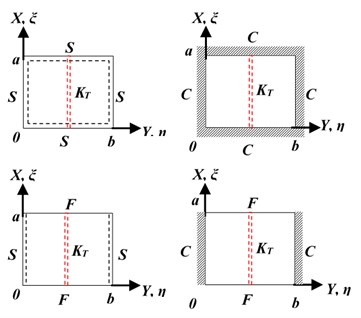## 3. Finite strip transition matrix (FSTM) with response surfaces (RS) method

### 3.1. Finite strip transition matrix (FSTM) method

In this approach, the plate is divided into $N$ space of strips between the ends of the plate as shown in Fig. 3. Each plate strips are considered as beam strip, and we can represent the displacement between individual strips by solve the shape function $\delta \left(\xi ,\eta ,\tau \right)$, this shape function can be assumed after normalized to non-Dimensional variables $\xi$ and $\eta$ depending on the unknown function ${Y}_{i}\left(\eta \right)$ to be determined and basic function ${X}_{i}\left(\xi \right)$ in $\xi$-direction, in the form:

5
$\delta \left(\xi ,\eta ,\tau \right)={\sum }_{i=0}^{N}{X}_{i}\left(\xi \right){Y}_{i}\left(\eta \right){e}^{i\omega \tau }.$

### 3.2. Response surface (RS) method

The RS is more dependent tool for quality engineering areas [18, 19]. The methodology of the RS have two benefits, the approximately of the inverse problems can be solved without take in your account the engineering modeling, and the approximately results of RS can be estimated using powerful statistical tools. In this estimation work, the methodology of the RS was applied to predict the $\mathrm{\Omega }$ from Eigen function. For widly applications of the RS, the functions for the approximately of the inverse problems are polynomials, in order to simplify. For the cases of quadratic polynomials, the RS is addressed as follow:

6
$y={\beta }_{0}+{\sum }_{j=1}^{k}{\beta }_{j}{x}_{j}+{\sum }_{j=1}^{k}{\beta }_{jj}{{x}_{j}}^{2}+{\sum }_{i=1}^{k-1}{\sum }_{j=i+1}^{k}{\beta }_{ij}{x}_{i}{x}_{j},$

where: $k$ is the number of strips. In this case, there are $N$ discrete longitudinal strips spanning (beam strips), $y$ is the estimation results of the $\mathrm{\Omega }$ by RS method and the coefficients $\beta$ are obtained with the least square errors method [18, 19]. The estimations of the $\mathrm{\Omega }$ by RS function in Eq. (6) is expressed as follow:

7
$\mathrm{\Omega }={\beta }_{0}+{\sum }_{j=1}^{N}{\beta }_{j}{S}_{j}+{\sum }_{j=1}^{N}{\beta }_{jj}{{S}_{j}}^{2}+{\sum }_{i=1}^{N-1}{\sum }_{j=i+1}^{N}{\beta }_{ij}{S}_{i}{S}_{j},$

where: ${S}_{i}$, ${S}_{j}$ are a standard eigenvalue can be obtained from the shape function $W\left(\xi ,\eta ,t\right)$ by FSTM, $i=$ 1, 2, 3,…, $N$, $j=$1, 2, 3,…, $M.$

Fig. 3FSTM model on plate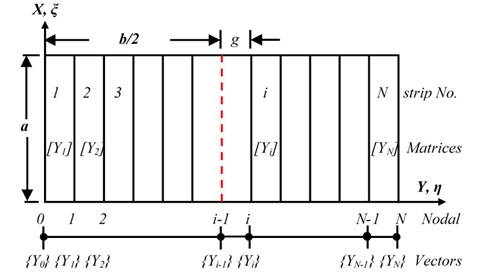In the case that varies $\mathrm{\Omega }$, the total number of parameters is $n$, the RS can be computed as follows using matrix expression:

8
$Y=X\beta +e,$

where:

$Y=\left\{\begin{array}{l}{y}_{1}\\ {y}_{2}\\ ⋮\\ {y}_{n}\end{array}\right\},X=\left[\begin{array}{lllll}1& {x}_{11}& {x}_{12}& \dots & {x}_{1k}\\ 1& {x}_{21}& {x}_{22}& \dots & {x}_{2k}\\ ⋮& ⋮& ⋮& \ddots & ⋮\\ 1& {x}_{n1}& {x}_{n2}& \dots & {x}_{nk}\end{array}\right],\beta =\left\{\begin{array}{l}{\beta }_{0}\\ {\beta }_{1}\\ ⋮\\ {\beta }_{k}\end{array}\right\},e=\left\{\begin{array}{l}{e}_{1}\\ {e}_{2}\\ ⋮\\ {e}_{n}\end{array}\right\},$

where: $e$ is an error vector. The unbiased estimator $b$ of the coefficient vector $\beta$ is obtained using the well-known least square error method as follows:

9
$b={\left({X}^{T}X\right)}^{-1}+{X}^{T}Y.$

The variance-covariance matrix of the $b$ is obtained as follows:

10
$Cov\left({b}_{i},{b}_{j}\right)={\sigma }^{2}{\left({X}^{T}X\right)}^{-1},$

where: the $\sigma$ is the error of $Y$. The estimated value of $\sigma$ is obtained as follows:

11
${\sigma }^{2}=\frac{S{S}_{E}}{n-k-1}.$

$S{S}_{E}$ is a square sum of errors, and expressed as follows:

12
$S{S}_{E}={Y}^{T}Y-{b}^{T}{X}^{T}Y.$

In order to judge the goodness of the approximation of the RS, the adjusted coefficient of multiple determination ${R}_{adj}^{2}$ is used:

13
${R}_{adj}^{2}=1-\left(\frac{S{S}_{E}}{\left(n-k-1\right)}/\frac{{S}_{yy}}{\left(n-1\right)}\right),$

where: ${S}_{yy}$ is the total sum of squares:

14
${S}_{yy}={Y}^{T}Y-\left({\left({\sum }_{i=1}^{n}{y}_{i}\right)}^{2}/n\right).$

The value of ${R}_{adj}^{2}$ is equal to or lower than 1.0. A higher value of ${R}_{adj}^{2}$ implies a better fit. When the RS shows a very good fit, ${R}_{adj}^{2}$ approaches 1.0. A good fit of the RS means that the RS gives good estimations for the FSTM technique used for the regression. Lower ${R}_{adj}^{2}$ values means poorer estimations and the error band of the estimated result is wider.

## 4. Results and discussion

In this part, the present method is used to estimate the natural frequency of the plate was presented in Fig. 1. The intermediate elastic line support at one direction and we can be expressed as follow, ${K}_{T}={T}_{b/2}{b}^{3}/{D}_{22}$, $T$ is translational stiffness. The plate model was designed of five symmetrically, angle-ply, laminates with the fiber angles $\left[\theta /-\theta /\theta /-\theta /\theta \right]$ is [45°/–45°/45º/–45°/45°] and has linear variable thickness, $\delta \left(y\right)$ as form $\delta \left(\eta \right)=1+\mathrm{\Delta }\eta$, $\mathrm{\Delta }={\delta }_{b}-{\delta }_{o}/{\delta }_{o}$ is the tapered ratio (see Fig. 1). The physical and mechanical properties of the basalt FRP laminate composite plate are shown in Table 1. The frequency parameter $\mathrm{\Omega }$ is evaluated in non-dimensional form, expressed as: $\mathrm{\Omega }={\left({m}_{o}t\left(\eta \right){\omega }^{2}{a}^{4}/{t}_{o}{D}_{22}\right)}^{1/2}$.

Table 1Physical and mechanical properties of the basalt FRP

 ${E}_{1}$ ${E}_{2}={E}_{3}$ ${G}_{1}={G}_{3}$ ${G}_{2}$ ${\upsilon }_{1}={\upsilon }_{3}$ ${\upsilon }_{2}$ $\rho$ 96.74 GPa 22.55 GPa 10.64 GPa 8.73 GPa 0.3 0.6 2700 kg/m3

### 4.1. The presented study verification

It is very important from the author to verify for the proposed method to decide whether his suggested method is suitable or not by compute the first six frequencies and compared with available results in literatures.

Table 2 shows a comparison between the first six frequency parameters from presented study and the corresponding results from literatures [5, 20-22]. literatures are used isotropic, square $\text{(}\beta =$1.0), uniform thickness ($\mathrm{\Delta }=$0) plates with a mid-line support in each direction, the physical and mechanical properties of the plate material are ${\upsilon }_{1}={\upsilon }_{3}=\text{0.3,}$${D}_{11}={D}_{22}=D=E{t}^{3}/\left[12\left(1-{\upsilon }^{2}\right)\right]\text{,}$${D}_{66}=\left(1-\upsilon \right)D/2\text{.}$ As results we can see a very close agreement is observed with a high value of adjusted coefficient ${R}_{adj}^{2}$.

Table 2Comparison between the first six frequency parameters Ω=ρtω2a4/D1/2

 ${R}_{adj}^{2}$ ${\mathrm{\Omega }}_{1}$ ${\mathrm{\Omega }}_{2}$ ${\mathrm{\Omega }}_{3}$ ${\mathrm{\Omega }}_{4}$ ${\mathrm{\Omega }}_{5}$ ${\mathrm{\Omega }}_{6}$ SSSS 78.957 94.590 94.590 108.240 197.392 197.392 Ref.  1.0 78.957 94.590 94.590 108.240 197.392 197.392 Ref  0.98 78.96 94.68 94.72 108.44 197.40 198.96 Ref  0.99 78.958 94.826 94.826 108.41 197.50 197.50 Ref  1.0 78.957 94.585 94.585 108.22 197.39 197.33 CCCC 108.229 127.417 127.417 144.109 242.818 242.778 Ref  1.0 108.299 127.417 127.417 144.109 242.818 243.778

### 4.2. The proposed technique results

Fig. 4 presents the amount of changes of the natural frequencies due to intermediate elastic support of laminated, the plate has the aspect ratio $\beta =$0.5 and tapered ratio $\mathrm{\Delta }=$ 0.5. As shown in the figure, for each frequency of six frequencies, we can observe the increases in the frequency with the increasing of the value of elastic restraint coefficient (${K}_{T}$)

Fig. 5 presents changes of the vibration behavior due to variation in the types of the plate ends (SSSS, CCCC, SSFF and CCFF). As shown in the figure, we can see the variation of the frequencies values, where, the plate with ends of fully clamped (CCCC) and semi-simply supported (SSFF) have been recorded the higher and lower values of the frequencies respectively, and the other types of the plate ends (SSSS and CCFF) are located in intermediate.

Fig. 6 represents the estimation results of the first six frequencies by RS. The ${R}_{adj}^{2}$ of estimation results are 0.999, 0.996, 0.987 and 0.964 for SSSS, CCCC, SSFF and CCFF plate respectively.

Fig. 4The relation between non-dimensional frequencies parameter (Ω) and elastic restraint coefficient (KT)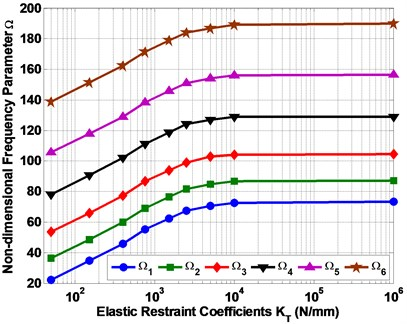Fig. 5The effect of the variation boundary conditions on non-dimensional frequencies parameter (Ω)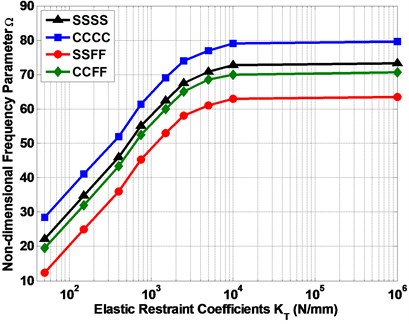All of the estimation results are plotted on the diagonal line (Fig. 6) to observe the performances of the present RS method to estimate non-dimensional frequencies ($\mathrm{\Omega }$). The error band is defined as the maximum error of the estimated $\mathrm{\Omega }$. The error band from the diagonal line is less than 0.01 for all types of boundary conditions. The results of the estimated $\mathrm{\Omega }$ is presented in Table 3. As a result, the RS gave good estimations for FSTM to calculate $\mathrm{\Omega }$ in composite plate.

Table 3The first six frequencies of Basalt FRP rectangular plate with intermediate elastic line support

 ${K}_{T}$ ${\mathrm{\Omega }}_{1}$ ${\mathrm{\Omega }}_{2}$ ${\mathrm{\Omega }}_{3}$ ${\mathrm{\Omega }}_{4}$ ${\mathrm{\Omega }}_{5}$ ${\mathrm{\Omega }}_{6}$ ${R}_{adj}^{2}$ SSSS 50 22.2 36.2 53.6 78.2 105.6 138.7 0.999 150 34.7 48.7 66 90.8 118 151.3 0.999 400 45.9 59.9 77.3 102 129 162.4 0.999 750 55.1 69.2 86.6 111.2 138.5 171.6 1.0 1500 62.5 76.5 94 118.6 146 179 1.0 2500 67.5 81.6 99 124 151 184 1.0 5000 70.8 84.9 103 127 154 187 1.0 10000 72.7 86.8 104 129 156.2 189 1.0 1E+06 73.2 87.2 104.6 129 156.6 190 1.0 CCCC 50 28.5 46.5 68.9 100.5 135.6 178 0.988 150 41 59 81.4 113 148 190.6 0.992 400 52 70 92.5 124 160 201 0.995 750 61.4 79.4 101.7 133.4 168.5 212 0.998 1500 69 87 110 141 176 218.5 0.999 2500 74 92 114 146 181 223.6 0.999 5000 77 95 117.6 149 184.3 227 1.0 10000 79 97 119.4 151 186.2 229 1.0 1E+06 79.5 97.5 120 151.5 187 229.4 1.0 SSFF 50 12.3 20.1 29.7 43.4 58.5 77 0.967 150 25 32.6 43 56 71 89.5 0.973 400 36 43.8 53.5 67 82.5 100.7 0.979 750 45.2 53.1 62.6 76.3 91.4 109.8 0.987 1500 53 60.5 70 83.8 99 117 0.991 2500 58 65.7 75 89 104 122 0.994 5000 61 69 78.6 92 107.6 125.6 0.997 10000 63 71 80 94 109 127.7 0.999 1E+06 63.5 71.4 81 94.8 109.5 128 1.0 CCFF 50 19.6 32 47.4 69.2 93.4 122.7 0.899 150 32 44.8 60 82 106 135.4 0.926 400 43.4 55.7 71 93 117 146.8 0.94 750 52.5 65 80.4 102.2 126.4 155.7 0.958 1500 60 72.7 88 110 133.8 163 0.972 2500 65 77.9 93 114.8 139 168 0.989 5000 68.6 81 96 118 142 171.7 0.999 10000 70 83 98 120 144 173.5 1.0 1E+06 70.6 83.4 98.7 121 144.6 174 1.0

Fig. 6The estimation results of non-dimensional frequency parameter Ω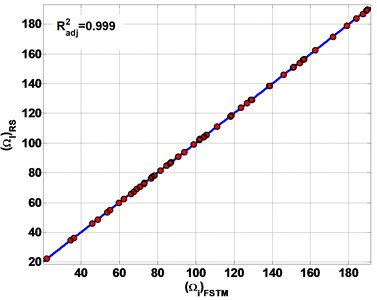a) SSSS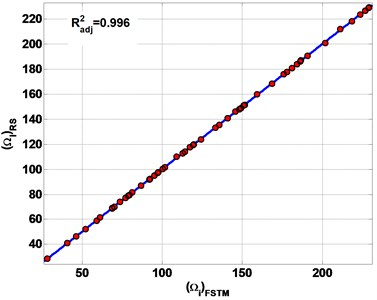b) CCCC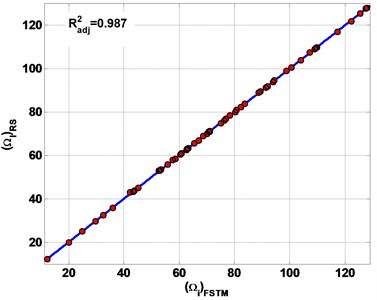c) SSFF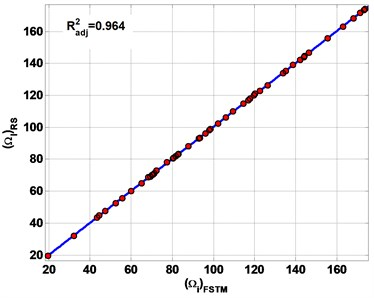d) CCFF

## 5. Conclusions

In the present study, the FSTM with RS approach is applied. The method has successfully estimation of the natural frequency ($\mathrm{\Omega }$) in one step of computing using the FSTM output of standard eigenvalue, in order to eliminate the iteration that implemented in FSTM algorithm to compute the natural frequency and decrease the error of estimation. Based on the FSTM results and the results estimated by RS, the following conclusions are drawn for basalt FRP laminated variable thickness rectangular plates with intermediate elastic support.

1) The RS data are provided excellent estimations of the natural frequency of basalt FRP plates for each case of boundary conditions and different ${K}_{T}$.

2) The increases in the frequency with the increasing of the value of elastic restraint coefficient (${K}_{T}$) is observed.

3) On other hand, the variation of the frequencies values, where, the plate with ends of fully clamped (CCCC) and semi-simply supported (SSFF) have been recorded the higher and lower values of the frequencies respectively, and the other types of the plate ends (SSSS and CCFF) are located in intermediate.

4) The RS technique could estimate $\mathrm{\Omega }$ with high adjusted coefficient ${R}_{adj}^{2}$ for ${K}_{T}=$50 are 0.999, 0.988, 0.967 and 0.899 for SSSS, CCCC, SSFF and CCFF plate respectively, for ${K}_{T}=$1E+06 are 1.0 for each boundary conditions.

5) It is observed that the adjusted coefficient ${R}_{adj}^{2}$ increases with increasing values of ${K}_{T}$ of intermediate elastic support.

6) The boundary condition SSSS has the highest performance of estimate $\mathrm{\Omega }$, the boundary condition CCFF has the lowest performance of estimate $\mathrm{\Omega }$, while the other boundary conditions are laid in between, with descending order of CCCC and SSFF respectively.

7) As a result, the method reveals excellent performance of estimations of natural frequencies.

8) Finally, author have revealed that the response surfaces are better than artificial neural networks for this inverse problem.

#### Cited by

Proceedings of the 4th International Conference on Advances in Civil and Ecological Engineering Research
Third International Conference on Computer Vision and Information Technology (CVIT 2022)
2022 International Conference on Electrical, Computer, Communications and Mechatronics Engineering (ICECCME)
2022 International Conference on Electrical, Computer, Communications and Mechatronics Engineering (ICECCME)
Proceedings of the 4th International Conference on Intelligent Science and Technology
Deep Learning-Based Crack Identification for Steel Pipelines by Extracting Features from 3D Shadow Modeling